## Example Questions

### Example Question #161 : Geometry

Quantity A

The perimeter of a square with a diagonal of 12 inches.

Quantity B

The perimeter of a rectangle with a diagonal of 10 inches and longer sides that are three times the length of its shorter sides.

The relationship cannot be determined from the information given.

Quantity A is greater.

The quantities are equal.

Quantity B is greater.

Quantity A is greater.

Explanation:

The diagonal of a square creates a 45-45-90 triangle; therefore, considering x as the value for the sides of the square in A, set up the ratio: 1/√2 = x/12 → x = 12/√2

Simplify the square root out of the denominator: x = (12√2)/2 = 6√2. The perimeter is equal to 4x; therefore, quantity A is 24√2.

Use the Pythagorean Theorem for B. We know that one side of the triangle is x and the other must be 3x. Furthermore, we know the hypotenuse is 10; therefore:

x2 + (3x)2 = 10→ x2 + 9x2 = 100 → 10x2 = 100 → x2 = 10 → x = √10.

Transform the √10 into √2  * √5 ; therefore, x = √2  * √5 . The perimeter of the rectangle will equal 3x + 3x + x + x = 8x = 8√2 * √5 .

Compare these two values. Quantity A has a coefficient of 24 in for its √2 . Quantity B has a coefficient of 8√5, which must be smaller than 24, because 8 * 3 = 24, but √5 is less than 3 (which would be √9 ); therefore, A is larger.

### Example Question #11 : Rectangles

A rectangle has a length that is twice that of its height. If the perimeter of that rectangle is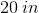, what is its area?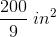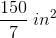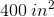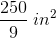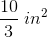Explanation:

Based on our first sentence, we know: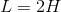The second sentence means: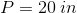, which is the same as: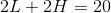Now, we can replacewith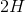in the second equation: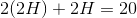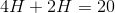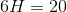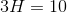Therefore,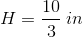Now, this means that: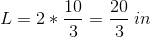If these are our values, then the area of the rectangle is: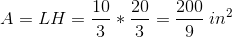### Example Question #163 : Geometry

A rectangle with an area of 64 square units is one-fourth as wide as it is long. What is the perimeter of the rectangle?Explanation:

Begin by setting up a ratio of width to length for the rectangle. We know that the width is one-fourth the length, therefore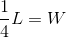.

Now, we can substitute W in the rectangle area formula with our new variable to solve for the length.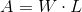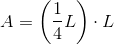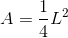Solve for length: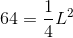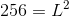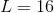Using the length, solve for Width: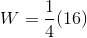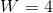Now, plug the length and width into the formula for the perimeter of a rectangle to solve: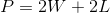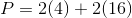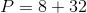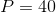The perimeter of the rectangle is 40.

Tired of practice problems?

Try live online GRE prep today.